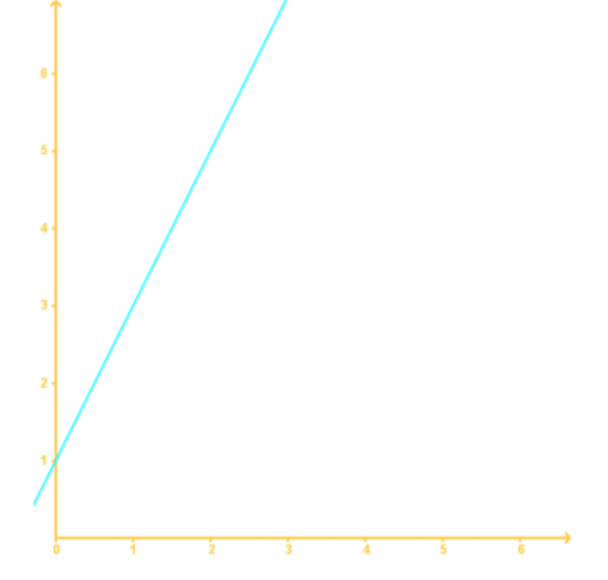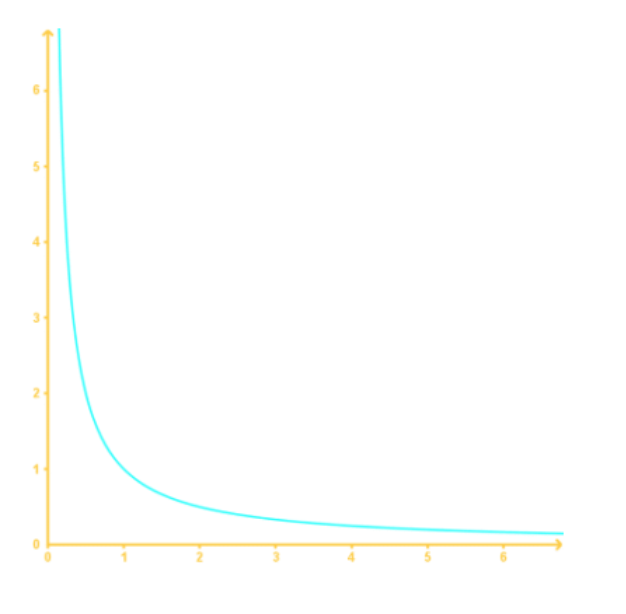# Inverse variation#### Everything You Need in One Place

Homework problems? Exam preparation? Trying to grasp a concept or just brushing up the basics? Our extensive help & practice library have got you covered.#### Learn and Practice With Ease

Our proven video lessons ease you through problems quickly, and you get tonnes of friendly practice on questions that trip students up on tests and finals.#### Instant and Unlimited Help

Our personalized learning platform enables you to instantly find the exact walkthrough to your specific type of question. Activate unlimited help now!##### Intros
###### Lessons
1. What is an Inverse Variation?
2. How to solve inverse variation problems?
##### Examples
###### Lessons
1. Identifying Indirect Variation Equations
Classify the following variations. (Direct or Indirect).
1. $b = -8a$
2. $m = \frac{n}{5}$
3. $p = \frac{-2}{q}$
4. $s = \frac{1}{7} \times t$
2. Identifying Indirect Variation Graphs
1.2.3. Evaluating Indirect Variations
Find the missing variable.
1. $y$ varies inversely with $x$. If $y=22$ when $x=-17$, find $y$ when $x=11$.
2. $y$ varies inversely with $x$. If $y=30$ when $x=18$, find $x$ when $y=5$.
4. Word Problem: Application of Indirect Variations
The time it takes to fly from Canada to China varies inversely as the speed of the plane. If the journey takes 13 hours at 950 km/h, how long would it take at 650 km/h?
###### Topic Notes

In this lesson, we will learn:

• Identifying Indirect Variation Equations
• Identifying Indirect Variation Graphs
• Evaluating Indirect Variations
• Word Problem: Application of Indirect Variations